##Involutive homographic relation

Here we consider involutive homographic relations x'=F(x) (i.e. coinciding with their inverse or equivalently F2=1). The general case is discussed in HomographicRelation.html . The case of involution happens when x' = (a*x + b)/(c*x -a). The involutions are distinguished by their fixed points, resulting by solving x = (a*x + b)/(c*x -a) or c*x2-2*a*x-b=0, with discriminant equal to disc=4*(a2+b*c). Below are two geometric interpretations of the involutions for the two relevant cases disc<0 (no fixed points) and disc>0 (two fixed points). The interpretation is by means of a circle with diameter points x and x' (centered on the line on which the involution is defined).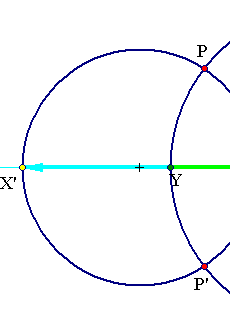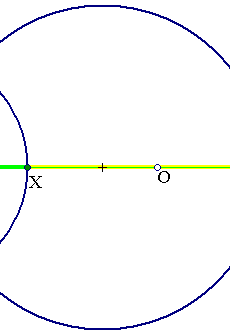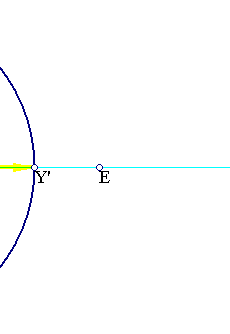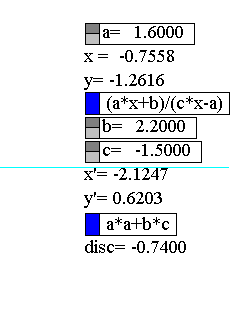The first case above is that of disc<0. The involution has no real fixed points, but has two complex conjugate P, P' fixed points. The circles with diameter x, x' build a circle bundle of intersecting type. All circles intersect at those two points P, P' resulting by solving the equation c*x2-2*a*x-b=0 in the complex domain.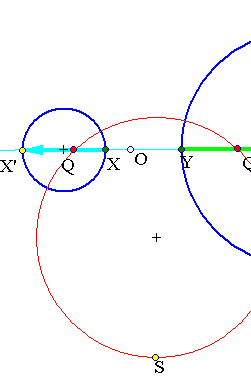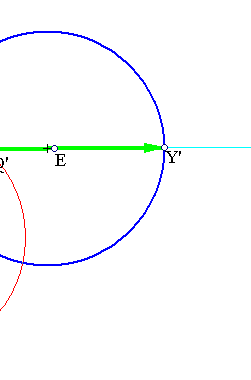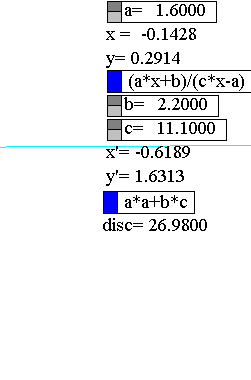The second case has disc>0. The involution has two real fixed points Q and Q', resulting by solving the equation c*x2-2*a*x-b=0. The circles with diameter x, x' build a circle bundle of non-intersecting type. Q and Q' are the limit points of this bundle. They can be determined as intersections of the line carying the involution with a single circle orthogonal to two circles of the bundle (in the figure the circle passing through the arbitrary point S).
There is an inverse to this procedure of definition of a circle bundle out of an involution. Having a bundle of circles and an arbitrary line one can define an involution induced by the bundle on the line. This is discussed in Involution2.html .
The deeper reason of the validity of this property is the Desargues Involution theorem, of which this is a particular case. In fact it is a particular case of that theorem applied to a circle bundle considered as a conic bundle of non-intersecting type (the member-conics are pairwise disjoint-see Remark). To prove it one has to go through the embedding of the bundle into the complex projective space. This is discussed in short in DesarguesInvolutionComplex.html .

Remark Note that two disjoint conics in the real projective plane become intersecting by embedding them into the complex projective plane. For example all circles of a circle bundle, embedded in the projective plane pass through the so-called cyclic-points, represented, in the standard model of projective plane by the tripples [1, i, 0], [i, 1, 0], (i) denoting the pure imaginary unit.

### See Also

CircleBundles.html
DesarguesInvolution.html
DesarguesInvolutionComplex.html
HomographicRelation.html
Involution2.html
Involution3.html

 Produced with EucliDraw© http://users.math.uoc.gr/~pamfilos/